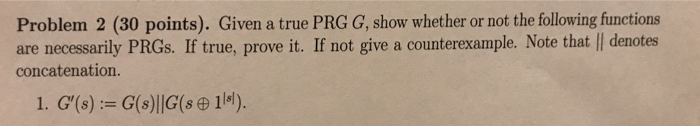# If A is a subset of B (not necessarily proper) and B is a subset of C (not necessarily proper) the A is a proper subset of C

If A is a subset of B (not necessarily proper) and B is a subset of C (not necessarily proper) the A is a proper subset of C.

True or False
if false must provide a counterexample

##### Add Answer of: If A is a subset of B (not necessarily proper) and B is a subset of C (not necessarily proper) the A is a proper subset of C
Similar Homework Help Questions
• ### If A is a subset of B (not necessarily proper) and B is a subset of C (not necessarily proper) the A is a proper subset of C

If A is a subset of B (not necessarily proper) and B is a subset of C (not necessarily proper) the A is a proper subset of C.True or Falseif false must provide a counterexample

• ### If A is a subset of B and B is a subset of C, can you conclude that A is a subset of C

If A is a subset of B and B is a subset of C, can you conclude that A is a subset of C? Can you conclude that A is a proper subset of C? If A is a proper subset of B and B is a proper subset of C, can you conclude that A is a subset of C? Can you conclude that A is a proper subset of C? If A is a subset of B and...

• ### Suppose B is proper subset of C

Suppose B is proper subset of C.If n(c)=8, what is the maximum number of elements n B?What is the least possible number of elements B?

• ### I have to make a true statement with this question: {All states west of the Rocky Mountains} {All states west of the Atlantic Ocean} I have to determine if the two statements are: Proper Subset is a subset of is not a subset of proper subset and is

I have to make a true statement with this question:{All states west of the Rocky Mountains} {All states west of theAtlantic Ocean}I have to determine if the two statements are: Proper Subsetis a subset ofis not a subset ofproper subset and is a subset of

• ### Given a true PRG G, show whether or not the following functions are necessarily PRGs. If true, pr...Given a true PRG G, show whether or not the following functions are necessarily PRGs. If true, prove it. If not give a counterexample. Note that jj denotes concatenation G'(s) : = G (s) II G (s    1 IsI  )   Problem 2 (30 points). Given a true PRG G, show whether or not the following functions are necessarily PRGs. If true, prove it. If not give a counterexample. Note that l| denotes concatenation. Problem 2 (30 points). Given a true...

• ### If A is a finite set and B is a proper subset of A, prove that |B| < |A|

If A is a finite set and B is a proper subset of A, prove that |B| < |A|. Hint: B is finite. What is the union of the disjoint sets B and A - B? Note: |A| = size of A

• ### If S is a bounded set of real numbers, and S contains Sub(S) and inf(S), then S is a closed...

Is this true or false? If true , provide a proof,; if false, provide a counterexample

• ### Describe whether the following statements are true or false and explain

Describe whether the following statements are true or false and explain. A. If n(A)<n(B), then A is a proper subset of B. B. If n(A) is less than or equal to n(B), then A is a subset of B. C. If A is a proper subset of B, then n(A)<n(B). D. If A is a proper subset of B, then n(A)is less than or equal to n(B). I say that the answer to A. is false but I'm not sure...

• ### Tell whether each of the following is true or false for all sets, A, B, or C

Tell whether each of the following is true or false for all sets, A, B, or C. If false give a counterexample. (A-B) U A= A(-B) U (B-A) i know its false but how would i do a counterexample?

• ### Part 1 I have to use symbols in my anwser Suppose B is proper subset of C If n(c)=8, what is the maxium number of elements in n (B) What is the least possible numbers of Elements in B

Part 1 I have to use symbols in my anwser Suppose B is proper subset of CIf n(c)=8, what is the maxium number of elements in n (B)What is the least possible numbers of Elements in B?Part 2Suppose C is a subset of D and D is a subset of CIf n (C)=5, find n (D) What is other relationship exists between sets C and D?

Need Online Homework Help?